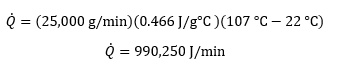## Calculating CFM of Air Needed for Cooling

It’s easy to know that EXAIR’s vortex tubes can be used to cool down parts and other items, but did you know that our air knifes can be used to cool down these same things? It’s the same process that we do every day to cool down hot food by blowing on it. Every molecule and atom can carry a set amount of energy which is denoted by physical property called Specific Heat (Cp); this value is the ration of energy usually in Joules divided by the mass multiplied by the temperature (J/g°C). Knowing this value for one can calculate the amount of air required to cool down the object.

Starting out you should note a few standard values for this rough calculation; these values are the specific heat of Air and the specific heat of the material. Using these values and the basic heat equation we can figure out what the amount of energy is required to cool. The specific heat for dry air at sea level is going to be 1.05 J/g*C which is a good starting point for a rough calculation; as for the specific heat of the material will vary depending on the material used and the composition of the material.Using the standard heat equation above add in your variables for the item that needs to be cooled down. In the example I will be using a steel bar that is 25 kg in mass rate and cooling it down from 149 °C to 107 °C. We know that the specific heat of steel is 0.466 J/g°C therefore we have everything needed to calculate out the heat load using air temperature of 22 °C.Using the heat rate, we can convert the value into watts of energy by multiplying the value by 0.0167 watts/(J/min) which gives us 16,537.18 watts. Furthermore, we can then convert our watts into Btu/hr which is a standard value used for cooling applications. Watts are converted into Btu/hr by multiplying by 3.41 Btu/hr/watt, giving us 56,391.77 Btu/hr.Once you have Btu/hr you can plug the information into a re-arranged Cooling power formula to get the amount of CFM of air required for cooling.As you can see in order to cool down this steel bar you only need to 343 CFM of air at 72°F. This can be done very easily and efficiently by using one of EXAIR’s Air Amplifiers or Air Knife. Sometimes you don’t need to use a vortex tube to cool down an object; sometimes simply blowing on it is good enough and its pretty simple to calculate out which product would fit your application the best.

If you have any questions about compressed air systems or want more information on any EXAIR’s of our products, give us a call, we have a team of Application Engineers ready to answer your questions and recommend a solution for your applications.

Application Engineer
EXAIR Corporation
Visit us on the Web